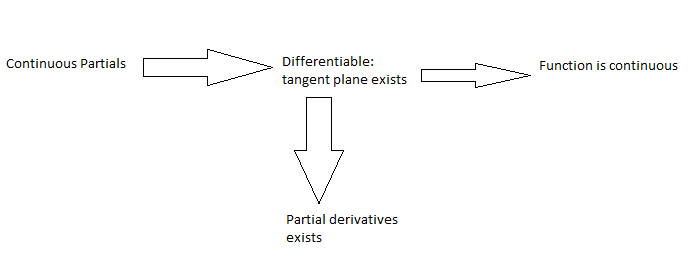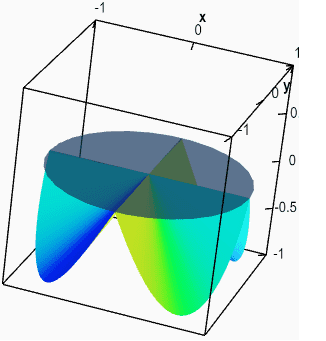# Differentiability of multivariable functions

• I
Sho Kano
What does it mean for a ##f(x,y)## to be differentiable at ##(a,b)##? Do I have to somehow show ##f(x,y)-f(a,b)-\nabla f(a,b)\cdot \left( x-a,y-b \right) =0 ##? To show the function is not though, it's enough to show, using the limit definition, that the partial derivative approaching in one direction is not equal to the partial from another direction right?

Last edited:

Homework Helper
Gold Member
Yes that is sufficient, but it is not necessary. A function may have both those partial derivatives exist and be equal, yet not be differentiable at that point.

Sho Kano
Yes that is sufficient, but it is not necessary. A function may have both those partial derivatives exist and be equal, yet not be differentiable at that point.
So to summarize, because ##f(x,y)## is a surface, to show that the function is diff at a point, we have to take into account all the partial derivatives in all directions at the point, and they all have to exist. Another option is to only look at the partial with respect to x or y, and then approach in different directions; if they are different, then the function is already not diff. However, if they are the same, it still does not guarantee diff because we can approach in infinitely many ways? Then that means currently, I can only disprove whether or not a function is diff. Is this where the "gradient" definition comes in?

Homework Helper
Gold Member
There is usually an easy way to prove differentiability, using the multidimensional differentiability theorem. All you need to do is show that both the ##x##-direction and ##y##-direction partial derivatives of ##f## exist and are continuous on an open set containing ##(a,b)##

Sho Kano
All you need to do is show that both the xxx-direction and yyy-direction partial derivatives of fff exist and are continuous on an open set containing (a,b)
What about all the other directions? I thought a tangent plane had to exist in order for ##f(x,y)## to be diff

Homework Helper
Gold Member
The tangent plane will exist if the criteria for that theorem are met. Consider the function ##f:\mathbb R^2\to\mathbb R## whose value is 0 except when ##x=y\neq 0##, in which case the value is 1.

##\frac{\partial f}{\partial x}(0,0)## and ##\frac{\partial f}{\partial y}(0,0)## both exist but ##f## does not satisfy the requirement that it be partial differentiable in the ##x## and ##y## directions throughout some open set containing (0,0), because any such set will contain a point ##(x,x)## at which neither the ##x## nor ##y## direction partial derivative exists.

Last edited:
Sho Kano
I don't understand, how do those partials exist? Here is a chart I saw in a video (arrow means "a" implies "b")Continuous partials as in a ##{ C }^{ 1 }## function. ##{ C }^{ 1 }## meaning only with respect to x and y, or all directions?

This function isn't ##{ C }^{ 1 }##, but is differentiable right? Meaning it implies continuity and existence of partial derivativesLast edited:
Homework Helper
Gold Member